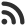Feed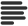Articles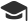Tutorials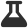CodingLab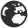CommunityLeaderboardFounder & Programmer at HackersFriend Updated Jan. 8, 2020, 12:05 a.m. ⋅ 71 views

# Print a matrix in spiral form

To print a matrix in spiral form we can use four loops.

Let's break down the problem, there 4 moves that are possible during spiral traversal.

• From left to right
• From top to bottom
• Right to left
• and From bottom to up

We'll need to have loop for each move. We'll loop around the entire matrix, with these moves and keep printing it each mode we visit.

Let's take an example to implement this apprach.

We are given an array, arr and we need to print it in spiral form, begining from the start index of the array.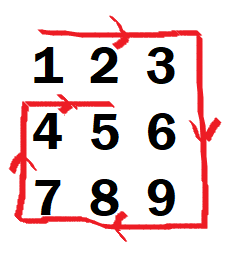Input

``````1 2 3
4 5 6
7 8 9``````

Output

``1 2 3 6 9 8 7 4 5``

Code

``````#include <bits/stdc++.h>
using namespace std;

void MatrixToSpiral(vector<vector<int>> matrix)
{
int i, k = 0, l = 0;
int m = matrix.size(); // last row index
int n = matrix.size(); // last column index

while (k < m && l < n) {
/* Print the first row from
the remaining rows */
for (i = l; i < n; ++i) {
cout << matrix[k][i] << " ";
}
k++;

/* Print the last column
from the remaining columns */
for (i = k; i < m; ++i) {
cout << matrix[i][n - 1] << " ";
}
n--;

/* Print the last row from
the remaining rows */
if (k < m) {
for (i = n - 1; i >= l; --i) {
cout << matrix[m - 1][i] << " ";
}
m--;
}

/* Print the first column from
the remaining columns */
if (l < n) {
for (i = m - 1; i >= k; --i) {
cout << matrix[i][l] << " ";
}
l++;
}
}
}

int main()
{
int rows, cols,temp;
cout<<"Enter the number of rows:";
cin>>rows;
cout<<"Enter the number of cols:";
cin>>cols;
cout<<"Enter elements for each row space separated";

vector<vector<int>> matrix;
for(int i=0; i<rows; i++)
{
vector<int> current_row;
for(int j = 0; j<cols; j++)
{
cin>>temp;
current_row.push_back(temp);
}
matrix.push_back(current_row);
}
MatrixToSpiral(matrix);
return 0;
}

``````
``````Enter the number of rows:3
Enter the number of cols:3
Enter elements for each row space separated1 2 3
4 5 6
7 8 9
1 2 3 6 9 8 7 4 5``````

Explanation

We have created a vector of vectors to store the matrix, and we are passing this to our function, which first prints from right to left, in the first for loop, then it prints elements from last column till the end then, it traverse in from right to left for the last raw, and then it traverses from bottom to top till the row-1 from which it started. Then this entire cycle continues.

 arrow_upward UpvoteComment arrow_downward downvote

Events

Nov. 28, 2018, 5:30 p.m.

Python from zero to hero

place Delhi

Aug. 13, 2018, 5:30 p.m.

Python from zero to hero

place Bangalore ( HackersFriend office BTM Layout)